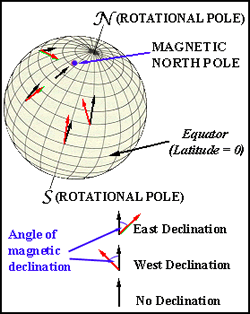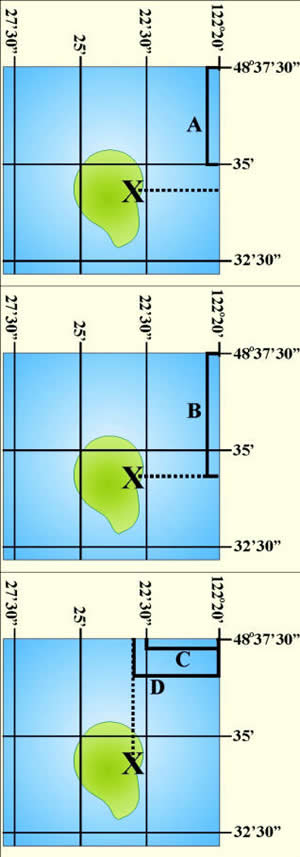-Stage 3.1-

Map Basics

These are some of the basics that you will need to be able to understand what a map is telling you and how to figure out some information on your own, like locations and distances.

The page is broken down into some of the various parts of a map:

Projections

Scales

Legend

North

Latitude and Longitude

Grid Systems

Previous Step

Next Step

Projections

What is a map?

A map is a scaled representation of the Earth's surface

What are the main problems with representing the Earth on a map?

1. The Earth is Round, a map is flat

2. The Earth is 3-D, a map is flat

How do we overcome these problems?

Different types of maps preserve different features of the Earth. A globe is the only perfect representation possible of the Earth. No map can preserve all features of a 3-D space on a 2-D map, but there are some properties that can be maintained:

Distance, Area, Scale, or Direction

Take a look here at the results of different projections on the globe.

Scales

What is a scale?

For a map to have any use at all, it must have a scale and the scale of the map must be consistent. The scale is how much smaller the map is than the Earth. It is usually listed in one of 3 ways:

Ratio Scale: 1:24,000

(1" on the map = 24,000" in real life, or 1cm = 24,000cm, or 1' = 24,000', etc)

Fractional Scale: 1/24,000

(Pretty much the same thing as a ratio scale)

Bar Scale: 0 |----------| 1

(A graphical representation of distance with each bar representing a particular type of measurement)

Legend

The legend on a map is where you find all the useful information on how to read the map, like the scale (mentioned above) and what different symbols mean. This legend is from the 2012 Michigan State Map.The main thing this type of legend (from a highway map) tells you:

• What the different types of roads are.
• The distance between 2 points:
• Whether along a highway or by using the scale bar
• What the other random map symbols mean like "rest area".

Most highway maps will only have a bar scale, not a fractional or ratio scale although it is easy to convert to these:

1 inch = 2.5 miles on this map

1 mile = 5280 feet = 63,360 inches

2.5 miles = 2.5*63,360 = 158,400 inches so the ratio scale is 1:158,400 or a fractional scale 1/158,400

No units are listed since they cancel out.

Bar scales are not always located in the legend and inserts will often have their own scales, since they are a different magnification than the rest of the map.

NorthThere are a 3 different types of North:

Geographic North - The North Pole. Also directly "up" on most maps, maps are most often made with geographic north towards the top edge of the map

Magnetic North - The direction your compass points. Since the magnetic north pole is not located at the same location as the geographic north pole (see picture to the right). Magnetic north is not a consistent thing so the date that is was mapped is always listed with the magnetic data (CUNY).

Grid North - Grid North is identified as the "North" for the particular grid system that is being used. The type of grid is listed on the map if there is one. Types of grids are described below.

Latitude and Longitude

Latitude and longitude are one of the hardest concepts to grasp in map reading. There are other locational systems used (as discussed below), but this is the most common. This is partially because when you become used to using it, you can usually picture the general area on the globe in your mind, unlike other techniques where you need to "decipher their code" before it is of any use.

Latitude - Lines parallel to the equator (labeled N-S)

Longitude - Lines that run from pole to pole (labeled E-W, starting with 0 through Greenwich, England)

Labeled - Degrees (°) Minutes (') Seconds (")

Degrees - There are 180 degrees in measuring latitude (90N to 90S)

There are 360 degrees in measuring longitude (180W to 180E)

Minutes - There are 60 minutes in a Degree

When measuring longitude, the closer to a pole you are, the closer the distance between degrees, minutes, and seconds

Seconds - There are 60 seconds in a Minute

Measuring Latitude and Longitude of a given point (X)Latitude:

1. Measure the distance between the first two intervals in the N-S direction [A]:

Distance A = 1.0625 inches

2. Count how many minutes occur in that interval:

Distance A = 48°35'00" - 48°37'30"

Distance A = 2 minutes 30 seconds

Distance A = 2.5 minutes

So now you have:

1.0625 inches

2.5 minutes

3. Measure the distance to the point you want to measure [B]:

Distance B = 1.327 inches

4. Now you want to determine B in decimal degrees:

So now you have:

1.327 inches

X minutes

5. Now calculate the distance in decimal degrees that occurs over that time by setting the 2 equations equal to each other then cross multiply:

1.0625 inches  = 1.327 inches

2.5 minutes            X minutes

1.0625 (X) = 3.3175

X = 3.122 minutes

6. Convert to minutes and seconds (if needed):

Multiply everything after the decimal by 60:

3.122 minutes = 3' 7.3"

7. Add the measured distance to the distance that you measure from in B:

(Since we measure down from the larger number we subtract from the top number)

48°37'30" - 3'7.3" = 48°34'22.7"

(All points in the US are labeled N (because north of equator) and W (because west of England))

Latitude = 48°34'22.7"N

Longitude:

9. Now do everything again in the E-W direction:

C = 122°20' - 120°22'30"

C = 2.5 minutes

C = 0.8125 inches

D = 0.9375 inches and X minutes

0.8125 inches = 0.9375 inches

2.5 minutes         X minutes

0.8125 (X) = 2.34375

X = 2.8846 minutes

X = 2'53.1"

X = 122°20' + 2'53.1"

X = 122°22'53.1"W

10. So now the complete latitude and longitude of X is:

(48°34'22.7"N, 122°22'53.1"W)

Grid Systems

Now as opposed to Latitude and Longitude, grid systems are used to "simplify" locating places. There a variety of different systems all with their own unique purposes. This is just 3 of the major ones.

UTM

Universal Transverse Mercator

This grid system was designed by the military to "metricize" the global location system.

On maps the UTM labels are blue and look like 3724000.

Overall this system is difficult to use and requires 2 charts to decipher what the seemingly random numbers mean.

PLSS

Public Land Survey System

Most states use this system and it is more commonly know as "Township and Range".

The system divides areas into blocks of 36 Townships.

These blocks are then broken down into 36 Sections.

Labeling is rather simple:

Townships run N-S (Labeled "T")

Ranges run E-W (Labeled "R")

The Township and Range block is then broken down into the 36 Sections running back and forth across the block. Any map with this system on it has a diagram showing how to label the sections. (Labeled "S")

Then sub sections are just based on quadrants (i.e. NW 1/4 S 36)

Labeling Example:

NW1/4, S36, T34N, R9W

SPCS

State Plane Coordinate System

Similar to PLSS except it covers the entire 50 states

The system was created to reduce errors in measurements.

It uses the black numbers running along the outsides of the maps and is a cross between UTM and PLSS.